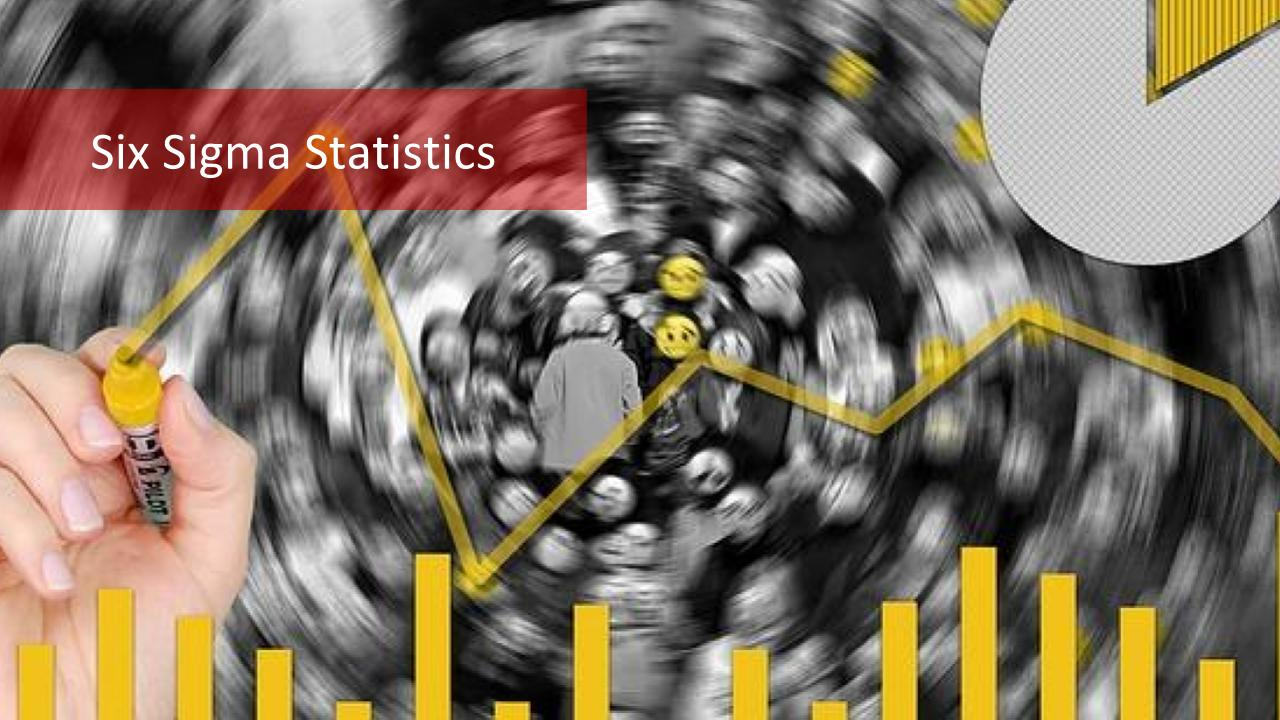### How Do The Six Sigma Statistics Work?

The Six Sigma approach is data-driven, therefore Six Sigma statistics play a large role in the Six Sigma problem-solving process. Six Sigma Green Belt training stresses the importance of using Six Sigma statistics to visualize and analyze the data collected for each metric that is important to the customer and that must be tightly controlled. […]### Six Sigma: What is the Normal Distribution Curve?

Six Sigma is a data-driven approach to problem-solving. Six Sigma approach involves many statistical and mathematical concepts such as the normal distribution curve. Lean Six Sigma courses discuss the main statistical concepts necessary to solve problems according to 6 sigma rules. Six Sigma principles rely heavily on the understanding of the normal distribution curve as […]